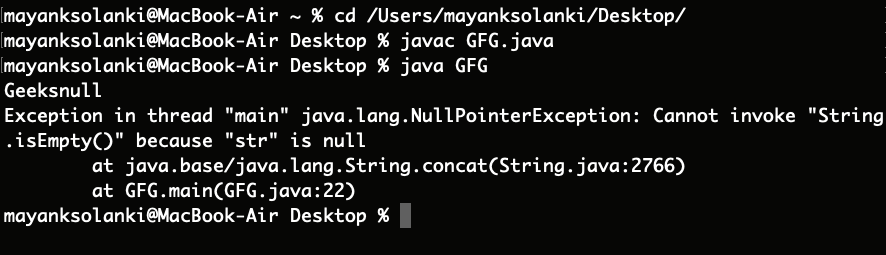Open In App

# Difference between concat() and + operator in Java

Strings are defined as an array of characters. The difference between a character array and a string is the string is terminated with a special character ‘\0’. Since arrays are immutable(cannot grow), Strings are immutable as well. Whenever a change to a String is made, an entirely new String is created. Concatenation is the process of joining end-to-end.

### concat() Method

The Java String concat() method of String class has been itself present inside java.util package concatenates one string to the end of another string. This method returns a string with the value of the string passed into the method, appended to the end of the string.

Illustration:

```Input:  String 1   : abc
String 2   : def
String n-1 : ...
String n   : xyz
Output: abcdef...xyz  ```

Example:

## Java

 `// Java Program to Demonstrate Working of concat() method` `// Main class``class` `GFG {` `    ``// Main driver method``    ``public` `static` `void` `main(String args[])``    ``{``        ``// Custom input string 1``        ``String s = ``"Geeks "``;` `        ``// Custom input string 2 is passed as in arguments``        ``// Here we are adding it to end of same string``        ``s = s.concat(``"for Geeks"``);` `        ``// Printing the concatenated string``        ``System.out.println(s);``    ``}``}`

Output

`Geeks for Geeks`

Now let us do well on the next concept where we will be discussing

### ‘+’ Operator

+ operator is used for concatenating strings on either side and is used just likely we are up to adding two numbers, henceforth providing us the flexibility to add on either side.

Example:

## Java

 `// Java Program to Demonstrate Working of + Operator``// over Strings` `// Main class``class` `GFG {` `    ``// Main driver method``    ``public` `static` `void` `main(String args[])``    ``{``        ``// Custom input strings``        ``String s1 = ``" Geeks "``;``        ``String s2 = ``" for Geeks "``;` `        ``// Now using + operator over string where``        ``// flexibility is provided to add on either side``        ``String s3 = s1 + s2;``        ``String s4 = s2 + s1;` `        ``// Printing strings formed after``        ``// using + operator``        ``System.out.println(s3);``        ``System.out.println(s4);``    ``}``}`

Output

``` Geeks  for Geeks
for Geeks  Geeks ```

Although concat() method of Strings class and + operator are both used for the concatenation of strings, there are some differences between them which are depicted below in the tabular format as follows:

Factor 1: Number of arguments the concat() method and + operator takes

• concat() method takes only one argument of string and concatenates it with other string.
• + operator takes any number of arguments and concatenates all the strings.

Example

## Java

 `// Java Program to Illustrate concat() method``// vs + operator in Strings` `// Main class``public` `class` `GFG {` `    ``// Main driver method``    ``public` `static` `void` `main(String[] args)``    ``{``        ``// Custom input string``        ``String s = ``"Geeks"``, t = ``"for"``, g = ``"geeks"``;` `        ``// Printing combined string using + operator``        ``System.out.println(s + t + g);` `        ``// Printing combined string using concat() method``        ``System.out.println(s.concat(t));``    ``}``}`

Output

```Geeksforgeeks
Geeksfor```

Factor 2: Type of arguments

• string concat() method takes only string arguments, if there is any other type is given in arguments then it will raise an error.
• + operator takes any type and converts to string type and then concatenates the strings.

Factor 3: concat() method raises java.lang.NullPointer Exception

• concat() method throws NullPointer Exception when a string is concatenated with null
• + operator did not raise any Exception when the string is concatenated with null.

Example

## Java

 `// Java Program to Illustrate Raise of NullPointer Exception``// in case of concat() Method` `// Main class``public` `class` `GFG {` `    ``// Main driver method``    ``public` `static` `void` `main(String[] args)``    ``{` `        ``// Input string 1``        ``String s = ``"Geeks"``;``        ``// Input string 2``        ``String r = ``null``;` `        ``// Combining above strings using + operator and``        ``//  printing resultant string``        ``System.out.println(s + r);` `        ``// Combining above strings using concat() method and``        ``// printing resultant string``        ``// It raises an NullPointer Exception``        ``System.out.println(s.concat(r));``    ``}``}`

Output:Factor 4: Creates a new String object

• concat() method takes concatenates two strings and returns a new string object only string length is greater than 0, otherwise, it returns the same object.
• + operator creates a new String object every time irrespective of the length of the string.

Example

## Java

 `// Java Program to Illustrate Creation of New String object``// in concat() method and + Operator` `// Main class``public` `class` `GFG {` `    ``// Main driver method``    ``public` `static` `void` `main(String[] args)``    ``{` `        ``// Input strings``        ``String s = ``"Geeks"``, g = ``""``;` `        ``// Using concat() method over strings``        ``String f = s.concat(g);` `        ``// Checking if both strings are equal` `        ``// Case 1``        ``if` `(f == s)` `            ``// Identical strings``            ``System.out.println(``"Both are same"``);` `        ``else` `            ``// Non-identical strings``            ``System.out.println(``"not same"``);` `        ``// Case 2``        ``String e = s + g;` `        ``// Again checking if both strings are equal``        ``if` `(e == s)` `            ``// Identical strings``            ``System.out.println(``"Both are same"``);``        ``else` `            ``// Non-identical strings``            ``System.out.println(``"not same"``);``    ``}``}`

Output

```Both are same
not same```

Factor 5: Performance

concat() method is better than the + operator because it creates a new object only when the string length is greater than zero(0) but the + operator always creates a new string irrespective of the length of the string.

Conclusion: From above two programs we draw see that both are somehow adding up two strings directly or indirectly. In concat method we are bound to while adding strings as we have to pass the string in the parameter while in other case of ‘+’ operator, it is simply adding up strings likely mathematics, so there is no bound we can add either side.

Below is the table to depict major differences between both the methods: